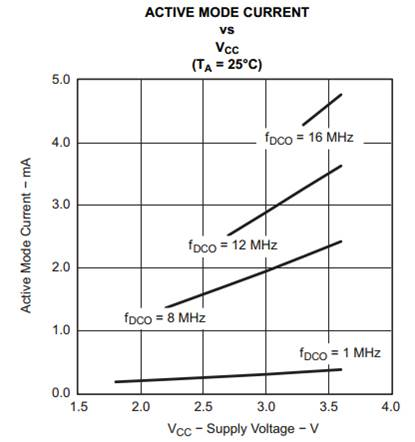# Embedded Basics – Linear Dynamic Current

When it comes time to start optimizing an embedded device for energy consumption it is often really tempting to start out by adjusting the operating voltage of the system.  If an engineer were to look back in his notes on MCU power consumption they would find something along the lines of

Power = Constant * Frequency * Voltage Squared + Current Load * Voltage

A quick look at this equation gets really exciting because with a voltage squared term we could save a lot of power by taking advantage of the exponential!  Or at least that is the way it looks.  When working with battery operated devices what the developer is really going after is current.  How can a systems current consumption be lowered?

Now this power equation still plays an important role in this endeavor.  Power after all is

Power = Current * Voltage / Time

Creating a quick substitution for power shows

Current * Voltage / Time =  Constant * Frequency * Voltage Squared + Current Load * Voltage

Simplifying so that the equation is representative of current over time results in

Current / Time =  Constant * Frequency * Voltage + Current Load

When optimizing for lowest power, the current draw of the load is not going to be something that can be adjusted.  That leaves the engineer with only two parameters than can be adjusted; a linear frequency relationship and a linear voltage relationship.  An excerpt from an MCU datasheet shows this linear relationship between current draw and supply voltage below:Reviewing the math and examining the plot reveals that adjusting the voltage supply does only decrease current consumption linearly and in fact only by a very little amount at best.  Other low power techniques may result in a far better energy savings then jumping straight to monkeying with the supply voltage.

This site uses Akismet to reduce spam. Learn how your comment data is processed.